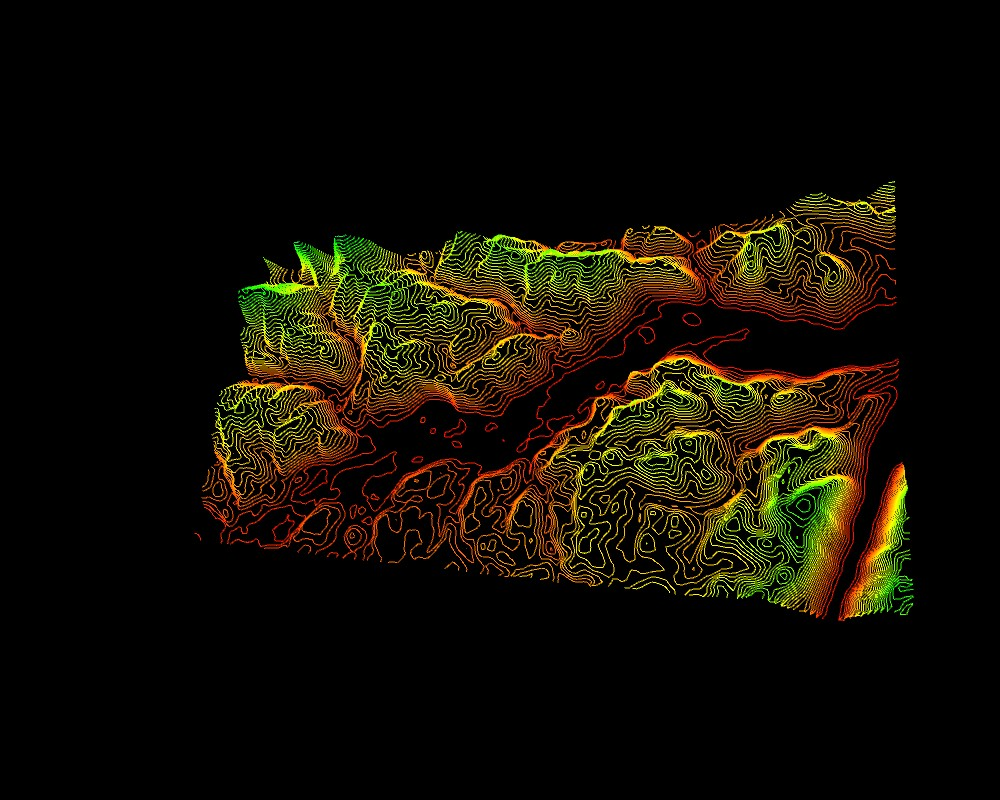## BlobDetection

A Library by Julien 'v3ga' Gachadoat for the Processing programming environment
Computer vision library for finding blobs in an image.## p5js

The library was converted to javascript for working with p5js environment. Check an example here.

# class EdgeVertex

This class is used by class BlobDetection to store points'coordinates when detecting edges of a blob. Its dimensions are normalized.

## Fields

float x, float y

Normalized coordinates of the vertex ( range [0;1] )

# class Blob

This class is used by class BlobDetection to store blob informations such as position, center. Its dimensions are normalized.

## Fields

float x, float y

Normalized coordinates of the blob's center ( range [0;1] )

float w, float h

Normalized dimensions of the blob ( range [0;1] )

float xMin, float yMin, float xMax, float yMax

Normalized min/max coordinates of the blob ( range [0;1] )

## Methods

int getEdgeNb()

Returns the number of edges for the blob.

EdgeVertex getEdgeVertexA (int iEdge)
EdgeVertex getEdgeVertexB (int iEdge)

Each detected blob maintains a list of edges. An edge is made of two points A and B (called edgeVertex). For a given index, these two functions enable you to access them. Both of them have normalized coordinates (in the range [0;1]). If the given index iEdge is not in the range [0;lineNumber-1], then the function returns null.

int getTriangleNb()

Each detected blob maintains a list of triangles (of class BlobTriangle) which is defining a "blob" polygon. The list is filled if computeTriangles() method of BlobDetection class was called at initialization.

BlobTriangle getTriangle(int iTriangle)

Returns a reference to the ith triangle of the list. If the index iTriangle is outside the range [0;triangleNumber-1], null is returned.

EdgeVertex getTriangleVertexA (BlobTriangle bTri)
EdgeVertex getTriangleVertexB (BlobTriangle bTri)
EdgeVertex getTriangleVertexC (BlobTriangle bTri)

These three functions allow you to access vertices that compose a triangle of a blob's polygon.

# class BlobTriangle

This class is used by class Blob to store informations on a single triangle of a blob's polygon.

## Fields

int iA, int iB, int iC

Indexes of EdgeVertices defining a triangle.

# class BlobDetection

## Methods

BlobDetection (int imgWidth, int imgHeight)

Constructor of the class. Paramaters are the dimensions of the image on which the detection is going to be applied.

static void setConstants(int blobMaxNb, int blobLinesMaxNb, int blobTrianglesMaxNb) Will set all the constants for memory allocation for differents objects used by all instances of BlobDetection class:
• blobMaxNb is the maximum number of blobs that an instance of BlobDetection can store. (default value is 1000)
• blobLinesMaxNb is the maximum number of edges ("lines") a single blob can store. (default value is 4000)
• blobTrianglesMaxNb is the maximum number of triangles a single blob can store. (default value is 500)
void computeTriangles()

A call to this function will tell the BlobDetection instance to store polygon informations of each detected blob. By default, polygons are not computed.

void setPosDiscrimination (boolean is)

If passed boolean value is true, the blob detection will attempt to find bright areas. Otherwise, it will detect dark areas (default mode). This setting is very useful when you decide to compute polygons for each blob.

void setThreshold (float value)

BlobDetection analyzes image for finding dark or bright areas, depending on the selected mode. The threshold is a float number between 0.0f and 1.0f used by the blobDetection to find blobs which contains pixels whose luminosity is inferior (for dark areas) or superior (for bright areas) to this value.

void activeCustomFilter(Object parent)

Make a call to this function to allow BlobDetection to fire an event each time a blob has been found by calling the function boolean newBlobDetectedEvent (Blob b), which has to be implemented in parent object passed to the BlobDetection. Inside the callback, a boolean value has to be returned: true means 'keep this blob', false means 'discard this blob'.

void computeBlobs (int[] pixels)

Compute the blobs in the image.

int getBlobNb ()

Returns the numbers of blobs detected in an image.

Blob getBlob (int n)

Returns the blob whose index is n in the list of blobs. Returns null if n is outside the range [0;blobNumber-1].

Processing 4.0b8

## Examples

Find a list of examples in the current distribution of BlobDetection